## 引言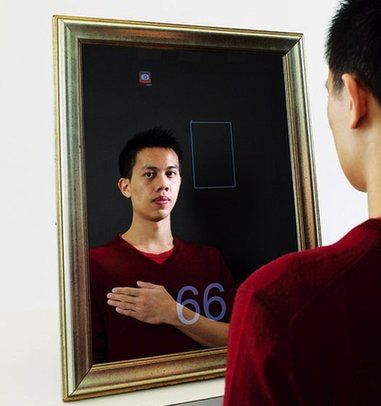Mingzhe Poh 的这面神奇的镜子的原理是利用了血液在人体内流动时光线的变化  ：心脏跳动时血液会通过血管，通过血管的血液量越大，被血液吸收的光线也越多，人皮肤表面反射的光线就越少。因此，通过对图像的时频分析就可以估算出心率。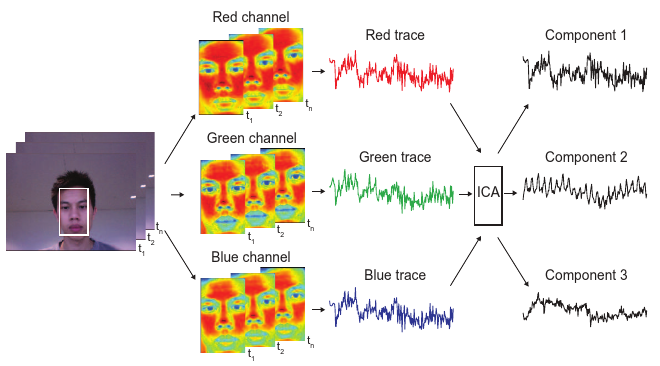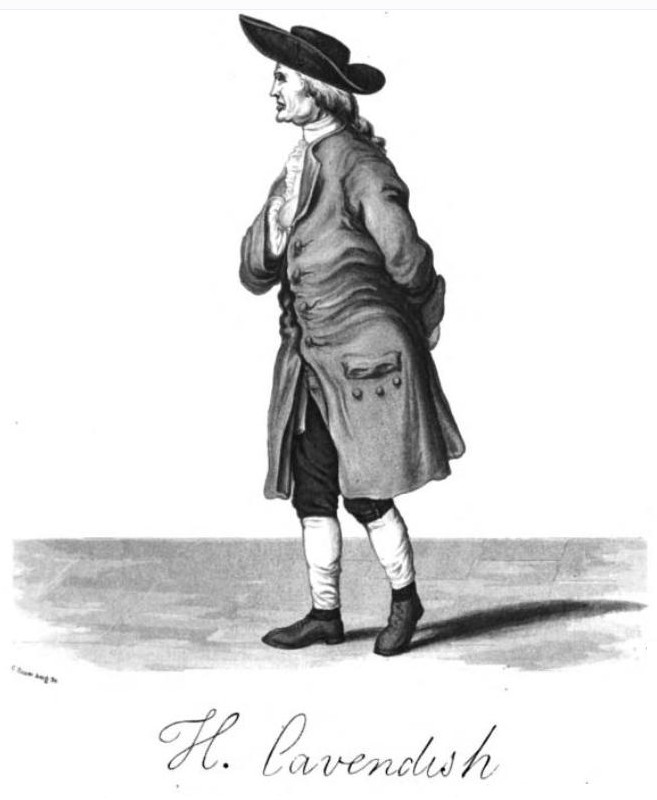附图 1 Henry Cavendish

## 最早的放大：卡文迪许扭秤实验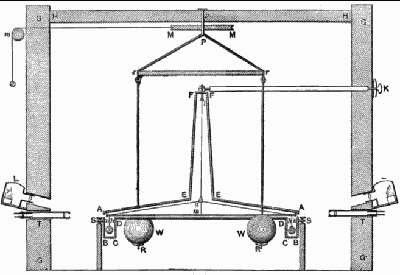1. 何为“变” —— 如何找出不易观察的微小变化量；
2. 放大“变” —— 如何放大这个变化量，使之肉眼可见。

## 拉格朗日视角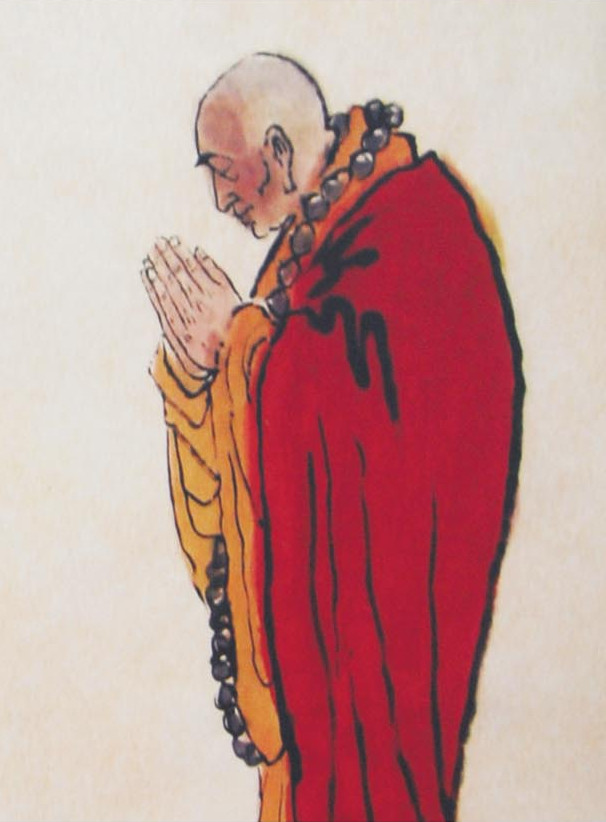附图 2 六祖慧能

1. 何为“变” —— 感兴趣的像素点随着时间的运动轨迹，这类像素点往往需要借助人工或其他先验知识来辅助确定；
2. 放大“变” —— 将这些像素点的运动幅度加大。

2005 年，Liu 等人最早提出了一种针对影像的动作放大技术，该方法首先对目标的特征点进行聚类，然后跟踪这些点随时间的运动轨迹，最后将这些点的运动幅度加大。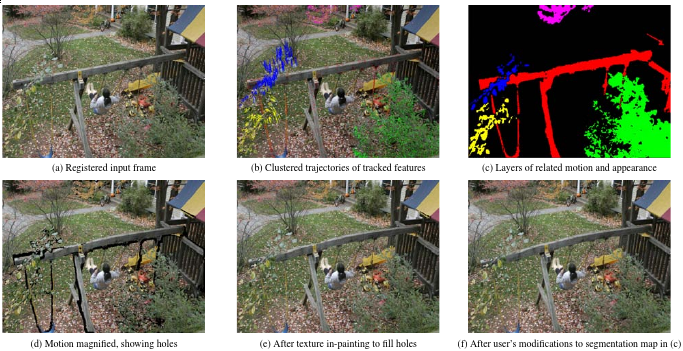• 需要对粒子的运动轨迹进行精确的跟踪和估计，需要耗费较多的计算资源；
• 对粒子的跟踪是独立进行的，缺乏对整体图像的考虑，容易出现图像没有闭合，从而影响放大后的效果；
• 对目标物体动作的放大就是修改粒子的运动轨迹，由于粒子的位置发生了变化，还需要对粒子原先的位置进行背景填充，同样会增加算法的复杂度。

## 欧拉视角

1. 何为“变” —— 整个场景都在变，而我们所感兴趣的变化信号藏在其中；
2. 放大“变” —— 通过信号处理手段，将感兴趣的信号分离，并进行增强。

2012 年， Wu 等人从这个视角着手，提出了一种称为欧拉影像放大技术（Eulerian Video Magnification）的方法，其流程如下：

1. 空间滤波。将视频序列进行金字塔多分辨率分解；
2. 时域滤波。对每个尺度的图像进行时域带通滤波，得到感兴趣的若干频带；
3. 放大滤波结果。对每个频带的信号用泰勒级数来差分逼近，线性放大逼近的结果；
4. 合成图像。合成经过放大后的图像。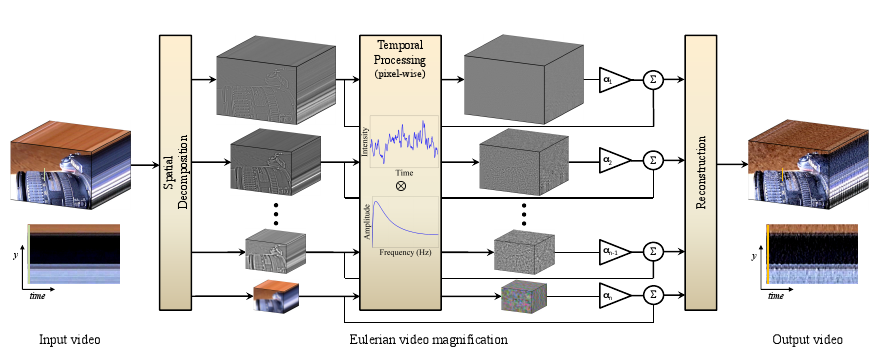## 算法细节

### 空间滤波

1. 有助于减少噪声。图像在不同空间频率下呈现出不同的SNR（信噪比）。一般来说，空间频率越低，信噪比反而越高。因此，为了防止失真，这些基带应该使用不同的放大倍数。最顶层的图像，即空间频率最低、信噪比最高的图像，可使用最大的放大倍数，下一层的放大倍数依次减小；
2. 便于对图像信号的逼近。空间频率较高的图像（如原视频图像）可能难以用泰勒级数展开来逼近。因为在这种情况下，逼近的结果就会出现混淆，直接放大就会出现明显失真1 1对于这种情况，论文通过引入一个空间波长下限值来减少失真。如果当前基带的空间波长小于这个下限值，就减少放大倍数。

### 时域滤波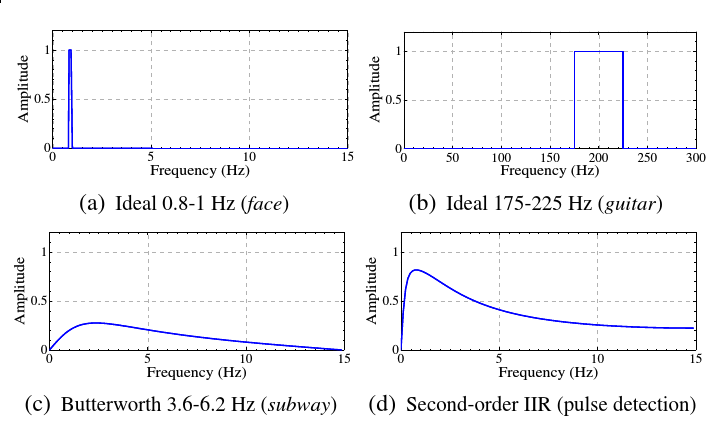### 放大和合成

eq: 1 »
\begin{aligned} I(x,t) & = f(x+\delta(t)) & , t >0 \\ I(x,0) & = f(x) & , t=0 \end{aligned}

eq: 2 »
$\hat{I}(x,t)=f(x+\color{red}{(1+\alpha)}\delta (t))$

eq: 3 »
$I(x,t)\approx f(x)+\color{blue}{\delta(t)\frac{\partial f(x)}{\partial x}}$

#### 理想情况

eq: 4 »
$B(x,t)=\color{blue}{\delta(t)\frac{\partial f(x)}{\partial x}}$

eq: 5 »
$\tilde{I}(x,t)=I(x,t)+\alpha B(x,t)$

eq: 6 »
$\tilde{I}(x,t)\approx f(x)+\color{red}{(1+\alpha)}\color{blue}{\delta(t)\frac{\partial f(x)}{\partial x}}$

eq: 7 »
$\tilde{I}(x,t)\approx f(x+\color{red}{(1+\alpha)}\delta(t))$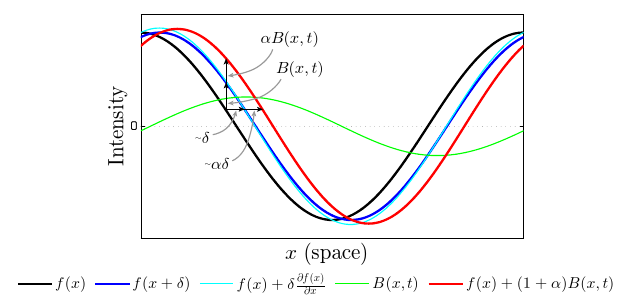#### 非理想情况

eq: 8 »
$B(x,t) = \sum_k \gamma_{k}\delta_{k}(t)\frac{\partial f(x)}{\partial x}$

eq: 9 »
$\tilde {I}(x,t)\approx f(x+\sum_k(1+\alpha_{k})\delta_{k}(t))$

### 放大倍数限制

eq: 10 »
$(1+\alpha)\delta(t) \lt \frac{\lambda}{8}$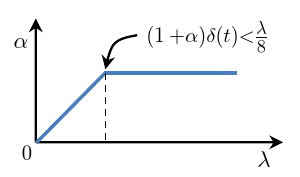## 算法实现

• 本文的源码遵守 LGPL v3 协议，但这个技术已由原作者申请了专利，因此请勿直接用于商业用途。
• 动作放大的模块引用了 Yusuke Tomoto 的 ofxEvm 项目的相关代码。这个项目基于 EVM 实现了对动作的放大。对于初学者，我建议先阅读他的代码。
• 另外特别感谢 Daniel RonAalessandro Gentilini 的大力援助，没有他们我无法完成这个程序。

## 结果

$\alpha$ $\lambda_c$ 频段 衰减因子

## 源码和程序

1. Poh, M.-Z., McDuff, D.J. and Picard, R.W. 2010. Non-contact, automated cardiac pulse measurements using video imaging and blind source separation. (2010).

2. Verkruysse, W., Svaasand, L.O. and Nelson, J.S. 2008. Remote plethysmographic imaging using ambient light. Optics express. 16, 26 (2008), 21434–21445.

3. Liu, C., Torralba, A., Freeman, W.T., Durand, F. and Adelson, E.H. 2005. Motion magnification. ACM Transactions on Graphics (TOG) (2005), 519–526.

4. Wu, H.-Y., Rubinstein, M., Shih, E., Guttag, J., Durand, F. and Freeman, W. 2012. Eulerian video magnification for revealing subtle changes in the world. ACM Transactions on Graphics (TOG). 31, 4 (2012), 65.

5. Wadhwa, N., Rubinstein, M., Durand, F. and Freeman, W.T. 2013. Phase-Based Video Motion Processing. ACM Trans. Graph. (Proceedings SIGGRAPH 2013). 32, 4 (2013).